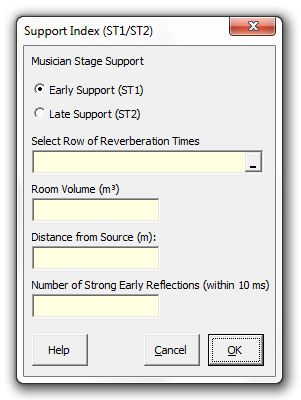### Strutt Help

Support Index (ST1/ST2)    1/1, 1/3

Strutt|Auditorium Acoustics|Stage Support Index (ST1/ST2) calculates the stage strutt parameters ST_1 and ST_2 using Barron's revised theory.Revised theory accounts for the decrease in the strength of the reflected sound with distance which occurs with distance in large(r) auditoria - i.e. the reverberant sound component is not constant when using revised theory, but decays exponentially with distance from the source.

The calculation assumes an omnidirectional point source and diffuse conditions in the auditorium.

The direct sound (up to 10 ms) is calculated using the following relationship:

d = (100(1+N))/r^2, where

r is the distance from the source.
'N' is the number of early reflections within 10 ms (e.g. stage reflection, if present)

Early reflections (from 20 ms up to 100 ms) are calculated using:

e=31200/V e^(-(0.04 r)/T) (e^(-(0.2764 r)/T) -e^(-0.1382/T)), where

V is the volume of the auditorium.
T is the reverberation time.

The late reflections (from 100 ms to 1000 ms) are calculated using:

l=31200/V e^(-(0.04 r)/T)(e^(-(0.1382 r)/T) -e^(-1.382/T))

Strutt calculates the strutt parameters as follows:

ST_1=10log(e/d)=10log((int_20^100 p^2(t)dt)/(int_0^10 p^2(t)dt))

ST_2=10log(l/d)=10log((int_100^1000 p^2(t)dt)/(int_0^10 p^2(t)dt))

References:
Barron Auditorium Acoustics and Architectural Design, 2nd edition, Appendix B.3
ISO 3381-1 (2009) Acoustics - Measurement of room acoustic parameters. Part 1: Performance spaces